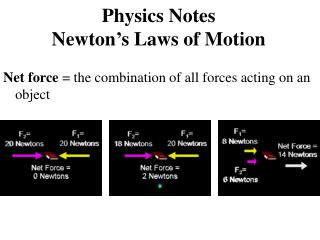# Physics Notes Newton’s Laws of Motion - PowerPoint PPT PresentationDownload PresentationPhysics Notes Newton’s Laws of Motion

Physics Notes Newton’s Laws of MotionDownload Presentation## Physics Notes Newton’s Laws of Motion

- - - - - - - - - - - - - - - - - - - - - - - - - - - E N D - - - - - - - - - - - - - - - - - - - - - - - - - - -
##### Presentation Transcript

1. Physics NotesNewton’s Laws of Motion Net force = the combination of all forces acting on an object

2. Balanced forces = produce no change in the motion of an object. Unbalanced forces = make objects start to move, speed up, slow down, or change direction.

3. In 1687, Isaac Newton published Philosophiae Naturalis Principia Mathematica. In this book he explained the relationship between force and motion. His three laws of motion can be used to explain the movement of all objects in the universe.

4. Newton’s First Law of Motion = An object at rest will stay at rest unless acted on by an unbalanced force. An object in motion will stay in motion at the same speed and in the same direction unless acted on by an unbalanced force.

5. Inertia = an object’s tendency to resist a change in motion. All objects have inertia. The greater the object’s mass, the greater its inertia and the larger the force needed to overcome inertia.

6. Newton’s Second Law of Motion = The acceleration of an object by a force is inversely proportional to the mass of the object and directly proportional to the force. Force (N) = mass (kg) x acceleration (m/s2) F = m a

7. a= F/m F = 0.49 N m = 0.05 kg Find the acceleration of the marble. F = 68.6 N m = 7.0 kg Find the acceleration of the bowling ball.

8. Air resistance Air resistance and falling objects The force of gravity if pulling down on the feather and coin. The force of air resistance is pushing up on the feather and coin. The net force of the feather and coin is equal to the force of air resistance subtracted from the force of gravity. Gravity

9. Falling objects don’t accelerate through their whole fall. Eventually, the force of air resistance pushing up against the object equals the force of gravity pulling down on the object. When that happens, the net force on the falling object becomes zero, and so the object stops accelerating. The final speed is called terminal speed. *example: raindrops reach terminal speed as they fall.

10. Newton’s Third Law of Motion = For every action, there is an equal and opposite reaction.

11. Momentum = depends on the object’s mass and velocity. The more momentum an object has, the harder it is to stop the object or change its direction. Momentum = mass x velocity p = m x v Kilogram-meters per second, kg·m/s (momentum) Kilograms, kg (mass) Meters per second, m/s (velocity)

12. Law of Conservation of Momentum States that any time objects collide, the total amount of momentum stays the same.